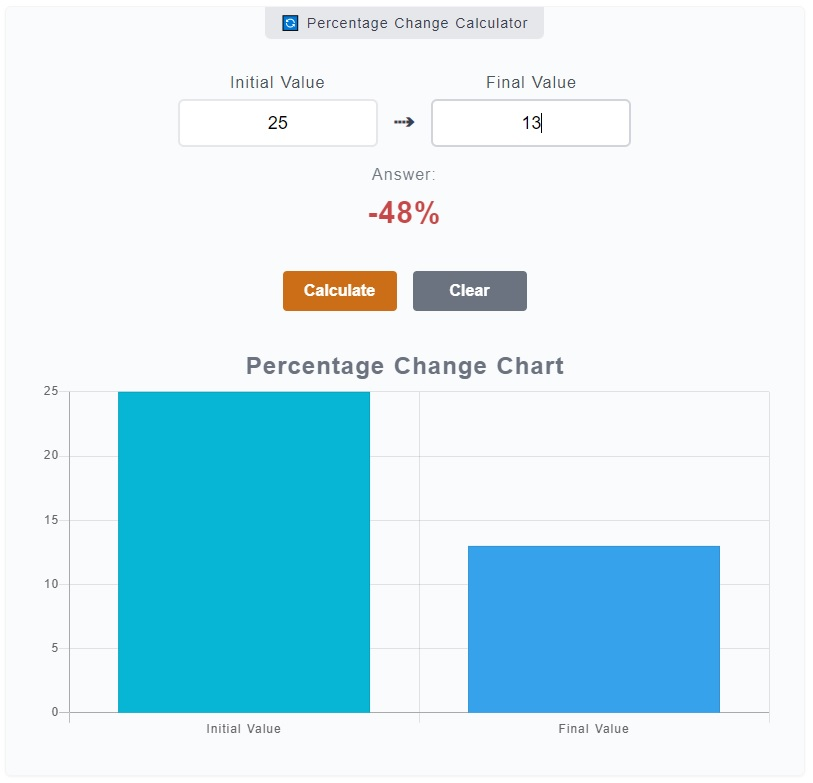# Percentage Change Calculator

The Percentage Change Calculator is used to measure the change of a number from an initial value to its final value in terms of percentage. A change of a value could be an increase, decrease or no change at all. Say you want to know the percentage change of a value from an initial value of `44` to the final value of `59`, or from `25` to `13`, this calculator will give you the percentage change.

🔄 Percentage Change Calculator

0%

## How to Use the Percentage Change Calculator

The `Percentage Increase Calculator` is quite a simple tool that you can see right away the input fields with corresponding labels. You can just enter numbers in them and click the `Calculate` button to get your answer.

If the text color of the answer is green, it means the value has increased. If it's red, the value has decreased. A bar chart also displays the graphical difference of initial value and final value. Here's a step by step guide to the calculator's usage:1. Step 1

On the calculator, there are two (2) input fields - initial value and final value. On the initial value, enter the number from its starting value. Enter the final value on the corresponding field as well. For instance, you want to know the percentage change from 44 to 49. Enter `44` on `Initial Value` input field and `49` to the `Final Value` input field.

2. Step 2

To get your results, you have two (2) options. You can press the `Enter` key on your keyboard or you can click on the `Calculate` button. To clear the input fields, result and percentage decrease chart, you just need to click the `Clear` button.